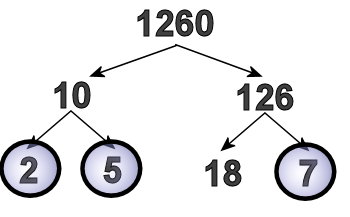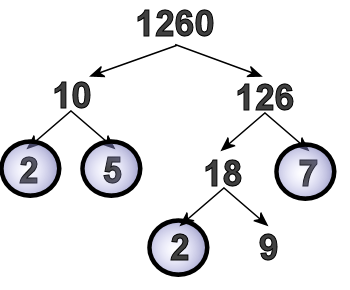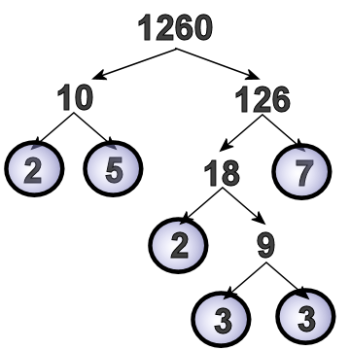Games
Problems
Go Pro!

Curtis asks us for an explanation for how to do a factor tree.

If a number isn't prime, then it can be written as a product of prime numbers. This product is called the "prime factorization." It's very useful to be able to find the prime factorizations of numbers, because that will help you determine things like the greatest common factor (GCF).

A factor tree is an easy way of finding the prime factorization of a number. So let's do a factor tree example. Let's say we want to find the prime factorization of the number 1,260. First, we ask ourselves, "Is there a pair of numbers that multiply together to make 1,260?"

That question has a lot of answers: 1,260 is 2 x 630, it's 3 x 420, it's 5 x 252, etc. When I did this, the first pair of numbers I spotted was 10 and 126. After all, if a number ends in a zero, like 1,260 does, we know it has to be a multiple of 10. So I drew a pair of arrows coming out of 1,260, and wrote 10 and 126 under the two arrows like this:I could have picked any of those other pairs, and it also would have worked fine.

Now that I've done this, I need to ask myself, "What is a pair of numbers that multiplies to 10?" and "What is a pair of numbers that multiplies to 126?"

For ten, I came up with two and five, so I drew arrows coming out of 10, and wrote two and five under them.

For 126, I came up with 7 and 18, so I drew arrows coming out of 126, and wrote 7 and 18 under them.

As I'm writing these, I realize that 2, 5, and 7 are all prime numbers (they can't be split up any more) so I draw circles around them.Some teachers, instead of having you circle the primes, will say, "Draw one arrow straight down from the two, and write two under it, so you keep bringing that two down to the bottom." Either way, you need to make sure you don't lose track of those prime branches!

Eighteen can split into factors; I chose to do 2 and 9. (I could have done 3 and 6 instead). So now it looks like this:We're almost done, because we've just got one number to split up (2 is prime, so we circle it, and then we just have to deal with the 9.)

Nine is three times three, so we make our arrows, and write two threes.

Since the threes are also prime, we'll circle those, and our prime factorization looks like this:Now that we've split 1,260 up into prime factors, we can say that 1,260 = 2 x 5 x 2 x 3 x 3 x 7.

But it would be better to write each prime factor only once, using exponents: 1,260 = 22 x 32 x 5 x 7.Like us on Facebook to get updates about new resources